• #include#include#include#include#define M 80//种群数量#define LEN 20//编码长度#define xmin -1//下限#define xmax 2//上限#define MMAX (int)pow(2,LEN)//编码长度对应的最大二进制数#define PI 3.1415926#...
#include#include#include#include#define M 80//种群数量#define LEN 20//编码长度#define xmin -1//下限#define xmax 2//上限#define MMAX (int)pow(2,LEN)//编码长度对应的最大二进制数#define PI 3.1415926#define PC 0.8//交叉概率#define PM 0.05//变异概率#define PD 0.2struct Node{int num,MyBinary[LEN];//num是对应二进制编码的整数值，MyBinary存放二进制编码double Myfitness;//Myfitness是适应度double Myfitsum;//Myfitsum是适应度占总体适应度的百分比，然后从第一个个体往后累加，主要用于选择操作}Nownode[M],Nextnode[M];//本代群体和下一代群体int nodeindex[M];//交叉时随机配对，存放配对的群体下标int T;double fx(double x)//被优化函数{double y;y=x*sin(10*PI*x)+2;//y=6-pow(x+6,2);//y=sin(0.7*x)/x;return y;}int randn(int temp)//产生0~MMAX之间的随机整数{return (int)(1.0*rand()/RAND_MAX*temp+0.5);}double double2double(struct Node node)//把对应的二进制数转化为对应区间的double数{return xmin+node.num*(xmax-xmin)/(pow(2,LEN)-1);}int calfitness()//计算适应度{int i;double temp,temp_sum=0,minfitness,maxfitness,avefitness;//minfitness作用是如果出现负的适应度，就做相应的变化double a,b,C=1.5;for(i=0;i{temp=double2double(Nownode[i]);Nownode[i].Myfitness=fx(temp);temp_sum+=Nownode[i].Myfitness;if(i==0){minfitness=Nownode[i].Myfitness;//i=0时，先给minfitness赋初值maxfitness=Nownode[i].Myfitness;}if(minfitness>Nownode[i].Myfitness){minfitness=Nownode[i].Myfitness;}if(maxfitness{maxfitness=Nownode[i].Myfitness;}}if(minfitness<0)//如果有负的适应度值，就把所以的适应度都加上一个数，使适应度全都为正数{temp_sum=0;for(i=0;i{Nownode[i].Myfitness+=-minfitness;temp_sum+=Nownode[i].Myfitness;}}//适应度线性变换avefitness=temp_sum/M;//计算平均适应度if(minfitness>(C*avefitness-maxfitness)/(C-1)){a=(C-1)*avefitness/(maxfitness-avefitness);b=(maxfitness-C*avefitness)*avefitness/(maxfitness-avefitness);}else{a=avefitness/(avefitness-minfitness);b=minfitness*avefitness/(avefitness-minfitness);}for(i=0;i{Nownode[i].Myfitness=a*Nownode[i].Myfitness+b;}Nownode.Myfitsum=Nownode.Myfitness;for(i=1;i{Nownode[i].Myfitsum=Nownode[i].Myfitness+Nownode[i-1].Myfitsum;//每一个Myfitsum都是自己的适应度加上前一个的Myfitsum}for(i=0;i{Nownode[i].Myfitsum=Nownode[i].Myfitsum/Nownode[M-1].Myfitsum;//每一个Myfitsum除以所有适应度之和，使Myfitsum为0~1之间}return 0;}int initpopulation()//初始化种群{int i,j,temp;for(i=0;i{temp=randn(MMAX);//产生0~MMAX之间的随机整数值Nownode[i].num=temp;//printf("%d\n",temp);for(j=LEN-1;j>=0;j--){Nownode[i].MyBinary[j]=temp%2;//给MyBinary赋值temp=temp/2;}}calfitness();//计算适应度return 0;}int assignment(struct Node *node1,struct Node *node2)//两个个体之间赋值操作，所以这里必须使用指针，{int j;for(j=0;j{node1->MyBinary[j]=node2->MyBinary[j];}node1->num=node2->num;node1->Myfitness=node2->Myfitness;node1->Myfitsum=node2->Myfitsum;return 0;}int copypopulation()//选择(复制)操作{int i,num=0;double temp;while(num{temp=1.0*rand()/RAND_MAX;//随机生成一个0~1之间的数for(i=1;i{if(temp>=Nownode[i-1].Myfitsum&&temp<=Nownode[i].Myfitsum){//Nextnode[num++]=Nownode[i];assignment(&Nextnode[num++],&Nownode[i]);//如果满足条件就赋值给下一代break;}}}for(i=0;i{//Nownode[i]=Nextnode[i];assignment(&Nownode[i],&Nextnode[i]);//更新本代个体}calfitness();//计算适应度return 0;}int isrepeat(int temp,int num)//交叉时要随机分组，防止出现重复的两个数，此函数检测是否下标重复{int i;for(i=0;i{if(nodeindex[i]==temp)return 1;}return 0;}int bin2int(struct Node *node)//把对应的编码转化为整数值{int j,num=0;;for(j=0;j{num+=(int)(pow(2,LEN-1-j)*(node->MyBinary[j]));}node->num=num;return num;}int crossposition(struct Node *node1,struct Node *node2,int p)//交叉操作，交叉点为p,参数必须是指针{int j,temp;for(j=LEN-1;j>=LEN-1-p;j--){temp=node1->MyBinary[j];node1->MyBinary[j]=node2->MyBinary[j];//交换两个个体的编码node2->MyBinary[j]=temp;}bin2int(node1);//交叉完成后更新num值bin2int(node2);return 1;}int crossover(){int i,temp;double pc_temp;for(i=0;i{do{temp=rand()%M;} while(isrepeat(temp,i));nodeindex[i]=temp;//首先产生了交叉的下标}for(i=0;i{temp=rand()%(LEN-1);pc_temp=1.0*rand()/RAND_MAX;if(pc_temp<=PC)//满足交叉条件就交叉{crossposition(&Nownode[nodeindex[i]],&Nownode[nodeindex[i+1]],temp);}}calfitness();//计算适应度return 1;}int mutation()//变异操作{int i,j;double pm_temp;for(i=0;i{for(j=0;j{pm_temp=1.0*rand()/RAND_MAX;if(pm_temp<=PM)//满足变异概率就进行变异操作{Nownode[i].MyBinary[j]=(Nownode[i].MyBinary[j]==0)?1:0;}}bin2int(&Nownode[i]);//更新个体的num值}calfitness();//计算适应度return 1;}int findmaxfit()//找到适应度最大的个体{int i,index=0;double temp=0;for(i=0;i{if(temp{index=i;temp=Nownode[i].Myfitness;}}return index;}int displaynode(){int i,j;printf("\n\n下标\tnum值\tx值\t        编码\t\tMyfitness\tMyfitsum\n");for(i=0;i{printf("第%d个\t%d\t%.3lf\t",i,Nownode[i].num,double2double(Nownode[i]));for(j=0;j{printf("%d",Nownode[i].MyBinary[j]);}printf("\t%.3lf\t\t%.3lf\n",Nownode[i].Myfitness,Nownode[i].Myfitsum);}return 1;}int main(){int index;int num=0,num1=0,num2=0;srand(time(NULL));while(num++<100){T=0;initpopulation();//初始化群体while(T++<=100){copypopulation();crossover();mutation();}index=findmaxfit();if(fabs(double2double(Nownode[index])-1.8)<=0.1){num1++;}else{num2++;}}printf("正确的结果次数有%d次\n",num1);printf("错误的结果次数有%d次\n",num2);return 0;}根据结果可知，计算结果正确的概率在98%以上。
展开全文• 问题1：遗传算法第一步就是要解决编码问题，常用二进制编码，用过matlab的都知道有自带的十进制转换二进制的API，但是生成的char类型变量却不方便完成后续计算适应度、交叉、变异等操作； 问题2：常见实现编码方式为...
问题介绍

问题1：遗传算法第一步就是要解决编码问题，常用二进制编码，用过matlab的都知道有自带的十进制转换二进制的API，但是生成的char类型变量却不方便完成后续计算适应度、交叉、变异等操作；
问题2：常见实现编码方式为先确定精度，根据目标精度反推最低需要的二进制编码位数然后编码，但是存在的问题是假如函数搜索范围是[-1,2]，需要0.001的精度，那么就是3000个数，需要12位二进制编码，但是完整的12位二进制编码可以表示到4096，如果直接用12位编码，那么将会在交叉及变异过程中，生成超出3000的数字，即超出搜索范围；

解决思路

针对问题1，必须将二进制编码转化为数组形式，才能实现单点交叉 变异等操作；
针对问题2，只能以补满位数为前提，即12位编码，在[-1,2]上分4096个数，精度即为0.0007；
本文拿解决如下函数，编码为例，理论求得最大值3.850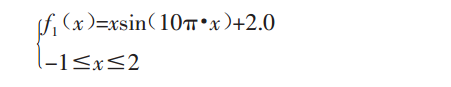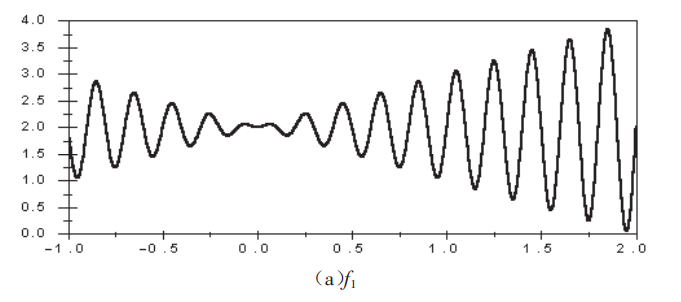具体实现

首先根据设定的编码位数，生成完整的二进制编码矩阵，利用matlab自带的dec2bin，然后根据转换出来的二进制的length与编码位数对比，将其补0满足设定的编码位数，以下是matlab代码实现% 将函数定义域转化为二进制，转化的实数按区间映射
% chromosome_size：设定的编码位数，例如本例采用12
% problem_size：完整二进制编码位数能表示的数值，如12位，能表示到2^12-1=4095
% problemDB：二进制编码矩阵
function[] =  ProblemDB_create()
global problem_size;
global chromosome_size;
global problemDB;
for i = 1:problem_size
arr = dec2bin(i);
for j = 1:length(arr)
problemDB(i,chromosome_size-length(arr)+j) = str2num(arr(1,j));
end
end
end


生成如下矩阵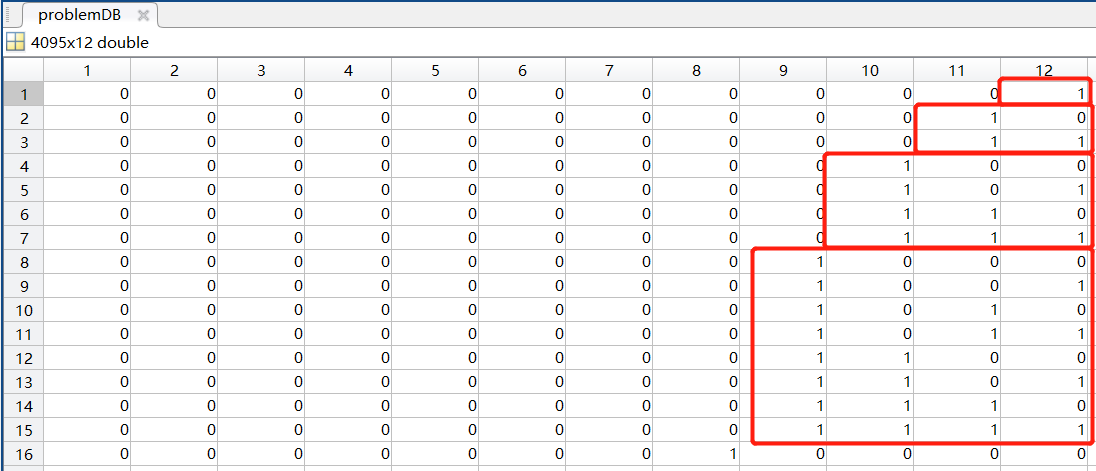生成了完整的编码，应该将其对应到函数的搜索区间上去，为了让代码可以复用，应该封装一个函数，可以提供输入区间起始和终止值，根据传入的基因编码计算对应在区间上的真值；具体思路也很简单，将二进制转换为10进制，计算其与完整二进制编码位数最大值的比例即可得知其映射在搜索区间上的真值；% 将二进制编码表示的个体转化为函数搜索区间上的实数值
% problem_size：同上
% indiv：个体的二进制编码数组
% from：搜索区间的起始值
% to：搜索区间的终止值
function[val] = realValue(indiv, from, to)
global problem_size;
dec = 0;
for i = 1:length(indiv)
if(indiv(1,i) == 1)
dec = dec + 2^(length(indiv)-i);
end
end
val = from + dec/problem_size*(to-from);
end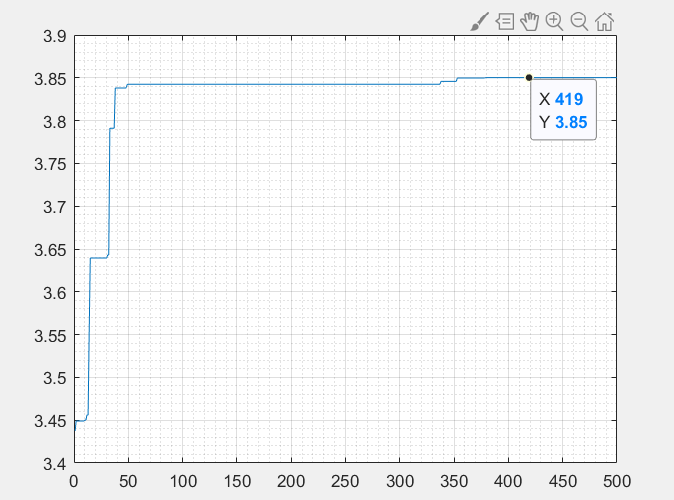总结

以上仅仅介绍了遗传算法解函数时的编码部分，交叉变异等其他步骤可以参考我上一篇博客，除了编码部分，修改下适应度函数和变异，其他基本相同，因此变量名都还是组卷的；
由于matlab里循环及数组索引需要从1开始，因此编码及搜索时并没有将0编进去，需要处理此问题的记得修改一下；


展开全文矩阵 matlab
• 转自： ... 用遗传算法求y=x*sin(10*pi*x)+2的最大值 -1= 精确到6位小数 pow(2,21)*1000000(2,22) 编码二进制长度为22 */ #include #in
转自： http://www.cnblogs.com/algorithms/archive/2012/05/19/2509322.html

 /*
用遗传算法求y=x*sin(10*pi*x)+2的最大值  -1=<x<=2
精确到6位小数
pow(2,21)<3*1000000<pow(2,22)
编码的二进制长度为22
*/
#include <stdio.h>
#include <string.h>
#include <stdlib.h>
#include <ctime>
#include <math.h>

#define N 3000000
#define PI 3.14159265
#define MAX(a,b) ((a)>(b)?(a):(b))

#define SIZE  50
#define MAXGEN  50
#define P_CORSS 0.75
#define P_MUTATION 0.05

#define LEN 22

typedef struct node
{
char x[LEN];
double fitness,fitsum;
}node;

node cur[SIZE],next[SIZE],max,min;

double randd()
{
return (double)rand()/RAND_MAX;
}
int randi(int k)
{
return (int)(randd()*k+0.5);
}

//计算当前种群中各个个体的适应度
void cal_fitness()
{
int i,j,k;
double d;
for(i=0;i<SIZE;i++)
{
k=0;
for(j=LEN-1;j>=0;j--)
k=(k<<1)+cur[i].x[j];
d=(double)k/N*3-1;
cur[i].fitness=d*sin(10*PI*d)+2;
cur[i].fitsum=i>0?(cur[i].fitness+cur[i-1].fitsum):(cur.fitness);
}
}

void init()
{
int tmp;
for(int i=0;i<SIZE;i++)
{
tmp=randi(N);
for(int j=0;j<LEN;j++)
{
cur[i].x[j]=tmp%2;
tmp=tmp>>1;
}
}
cal_fitness();
}

int sel()  //选择
{
double p=randd();
double sum=cur[SIZE-1].fitsum;
for(int i=0;i<SIZE;i++)
{
if(cur[i].fitsum/sum>p) return i;
}
}

//换代
void tran()
{
int i,j,pos;
//找当前种群最优个体
max=cur;
for(i=1;i<SIZE-1;i++)
{
if(cur[i].fitness>max.fitness)  max=cur[i];
}
for(int k=0;k<SIZE;k+=2)
{
//选择交叉个体
i=sel();
j=sel();

//选择交叉位置
pos=randi(LEN-1);

//交叉
if(randd()<P_CORSS)
{
memcpy(next[k].x,cur[i].x,pos);
memcpy(next[k].x+pos,cur[j].x+pos,LEN-pos);

memcpy(next[k+1].x,cur[j].x,pos);
memcpy(next[k+1].x+pos,cur[i].x+pos,LEN-pos);
}
else
{
memcpy(next[k].x,cur[i].x,LEN);
memcpy(next[k+1].x,cur[j].x,LEN);
}
//变异
if(randd()<P_MUTATION)
{
pos=randi(LEN-1);
next[k].x[pos]^=next[k].x[pos];

pos=randi(LEN-1);
next[k+1].x[pos]^=next[k+1].x[pos];
}
}
//找下一代的最差个体
min=next,j=0;
for(i=1;i<SIZE-1;i++)
{
if(next[i].fitness<min.fitness)  min=next[i],j=i;
}
//用上一代的最优个体替换下一代的最差个体
next[j]=max;

memcpy(cur,next,sizeof(cur));

cal_fitness();
}

//打印个体适应度和二进制编码
void print(node tmp)
{
printf("%.6lf",tmp.fitness);
for(int i=0;i<LEN;i++)  printf(" %d",tmp.x[i]);
printf("\n");
}

//打印种群
void printcur()
{
for(int i=0;i<SIZE;i++)
print(cur[i]);
}

void GA()
{
int cnt=0;
double ans;
while(cnt++<MAXGEN)
{
tran();
}
ans=cur.fitness;
for(int i=1;i<SIZE;i++)
ans=MAX(ans,cur[i].fitness);
printf("%.6lf\n",ans);
}

int main()
{
srand((unsigned)time(NULL));

init();
GA();

system("pause");
return 0;
}
展开全文• ## 遗传算法之二进制编码

万次阅读 热门讨论 2017-09-05 19:33:43
遗传算法的基本步骤遗传算法 GA 的流程如图所示：Created with Raphaël 2.1.0编码把所需要选择的特征进行编号，每一个特征就是一个基因，一个解就是一串基因的组合。为了减少组合数量，在图像中进行分块，然后把每...
遗传算法的基本步骤
遗传算法 GA 的流程如图所示：
Created with Raphaël 2.2.0
编码
把所需要选择的特征进行编号，每一个特征就是一个基因，一个解就是一串基因的组合。为了减少组合数量，在图像中进行分块，然后把每一块看成一个基因进行组合优化的计算。每个解得基因数量是要通过实验确定的。
遗传算法不能直接处理问题空间的参数，必须把它们转换成遗传空间的由基因按一定结构组成的染色体或个体。这一转换操作就叫做编码。评估编码策略常采用以下 3 个规范。（1） 完备性（Completeness）： 问题空间中的所有点（候选解）都能作为 GA 空间中的点（染色体）表现。（2） 健全性（Soundness）： GA 空间中的染色体能对应所有空间中的候选解。（3） 非冗余性（Nonredundancy）： 染色体和候选解一一对应。
目前几种常用的编码技术有二进制编码、浮点数编码、字符编码、编程编码等。二进制编码是遗传算法中最常见的编码方法，即由二进制字符集 {0， 1} 产生通常的 0, 1 字符串来表示问题的候选解。它具有以下特点：
（1） 简单易行；
（2） 符合最小字符集编码原则；
（3） 便于用模式定理进行分析。
染色体编码最常用的是二进制编码，对于离散性变量直接进行编码，对于连续性变量先离散化后再编码。
科普1：SPSS常用的基础操作（2）——连续变量离散化
（下面的这个地址中详细介绍了什么是连续变量离散化及其必要性）
人人都是数据咖：http://www.ppvke.com/Blog/archives/44271

科普2：连续特征的离散化：在什么情况下将连续的特征离散化后可以获得更好的效果？
问答来源于知乎：https://www.zhihu.com/question/31989952

举个例子
已知一元函数：
F(x) = xsin(10pi*x)+2      x∈[-1, 2]
现在要求在既定的区间内找出函数的最大值。
首先我们可以先用 MATLAB 把该函数的图像画出来：
clc, clear;
syms f(x); % 声明函数
x = linspace(-1,2,3000); % 定义 x, x 属于 [-1, 2]
f = x.*sin(10*pi.*x)+2; % 定义函数，因为 x 是向量，所以采用点乘
plot(f); % 画图

然后做出图形如下：
￼￼￼￼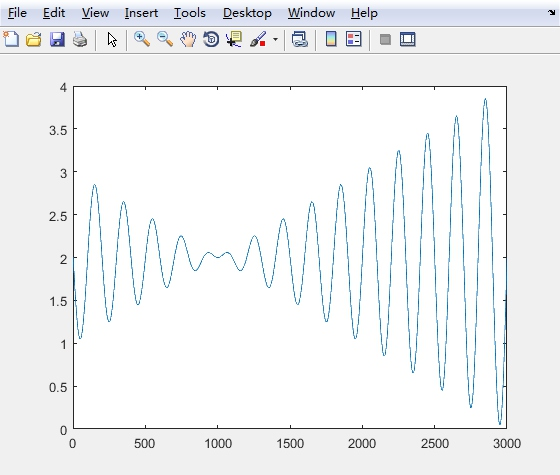我们可以看到，该图形显示说明该函数具有多个局部最优解，所以适合用遗传算法进行求解。
由遗传算法的基本步骤可知我们第一步应该是编码：
二进制编码
受到人类染色体结构的启发，我们可以设想一下，假设目前只有 0 和 1 两种碱基，我们也用一条链把它们有序的串连在一起，因为每一个单位都能表现出 1bit 的信息量，所以一条足够长的染色体就能为我们勾勒出一个个体特征的所有特征。这就是二进制编码
下面将介绍如何建立二进制编码到一个实数的映射。
明显地，一定长度的二进制编码序列，只能表示一定精度的浮点数。譬如我们要求小数点后精确到六位小数，由于区间长度为
2 - (-1) = 3

为了保证精度的要求，至少把区间 [-1, 2] 分为 3*10^6 等份。又因为
2097152=2^21 < 3*10^6 < 2^22=4194304

所以编码的二进制串至少有 22 位。
把一个二进制串 （b1b2…bn） 转换为区间里面对应的实数值通过下面两个步骤。
（1） 将一个二进制串代表的二进制转换为十进制数：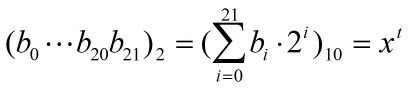（2） 对应区间的实数：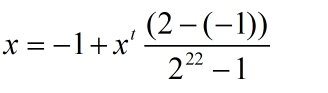或许有人并不知道是如何把数值转换到对应区间的实数的，为什么要这么做？我也是问了一下我的小伙伴才知晓的，下面我来简单的说一下：
通常我们归一化到 [0, 1]，有时候我们需要归一化到其它区间，这样算一下就可以找到数列的最小值 m 及最大值 M，如果指定的区间是 [a, b]，即：
m-->a, M-->b；

系数为：
k = (b-a)/(M-m)

对任意项Xn：变成：
X = a+k(Xn-m)

（二进制编码， 很多朋友评论说代码有问题，各位可以帮忙检查一下问题所在，嘻嘻~）
将十进制转换为二进制的 MATLAB 程序代码如下：
%% 将十进制数转换为二进制数（二进制编码）
% @params dec_num 十进制数
% @params N       需保留的二进制小数位数
function bin_num = encode(dec_num, N)
split = '.';
if rem(dec_num, 1) == 0
bin_num = dec2bin(dec_num);
float_num = zeros(1, N);
bin_num = strcat(num2str(bin_num), split);
bin_num = strcat(bin_num, num2str(float_num));
else
remainder = rem(dec_num, 1); % 小数部分
integer = floor(dec_num); % 整数部分
bin_num_int = dec2bin(integer);
i = 1;
flag = true;
while(flag == true)
remainder = remainder*2;
if (i > N) % 是否满足精度
return;
end
if remainder > 1
record(i) = 1;
remainder = rem(remainder, 1);
else if remainder == 1
record(i) = 1;
remainder = rem(remainder, 1);
else
record(i) = 0;
end
i = i+1;
end
bin_num_flt = record;
bin_num = strcat(num2str(bin_num_int), split);
bin_num = strcat(bin_num, num2str(bin_num_flt));
end
end
end

未完…（有机会再出第二章）


展开全文算法 优化
• Matlab实现遗传算法二进制编码）重点交叉crossover算法
• 假设我们要用遗传算法求解某个函数的最大值，x的取值范围是[-3.0,12.1]，那么我们现在就是要从这个取值范围内取出一些x来作为种群的个体，要取出多少个x这取决于你想要种群有多少个体，即种群规模。 如果我们用十...
• 今天小编就为大家分享一篇Python实现遗传算法(二进制编码)求函数最优值方式，具有很好的参考价值，希望对大家有所帮助。一起跟随小编过来看看吧
• 使用Matlab实现二进制编码方案的遗传算法，算法分为初始化init.m、编码encoding、解码decoding、交叉crossover、变异mutation、选择selection以及适应度函数的计算。该文件为初始化算法
•图像增强
• #include #include #include #include #define M 80 //种群数量 #define LEN 20 //编码长度 #define xmin -1 //下限 ...#define MMAX (int)pow(2,LEN)//编码长度对应的最大二进制数 #define PI 3.1415926 #c语言 struct
• 用matlab编写的经典二进制编码遗传算法算例,求函数最大值并画图，附带注释。
• 人工智能作业，使用二进制编码遗传进化算法解决TSP问题。
• 研究了遗传算法在寻找函数最优值方面的应用,比较分析了二进制遗传算法和八进制算法的函数优化结果。计算机仿真结果表明二进制编码遗传算法在函数优化中要优于八进制编码遗传算法
• 遗传算法的python实现（二进制编码），适用于python3.x环境，有详细的注释和两个给出的测试函数。
• 遗传算法编码方法各种各样，但二进制编码方式是最经典的一种，那么它的编码和解码该如何进行呢？或许本博客能给你一个具有参考价值的答案。 编码 经典遗传算法中使用“染色体”来代指个体，它由二进制串组成，...
• 本程序采用的是二进制编码精确到小数点后五位，经过计算可知对于 x 1 x_1 其编码长度为18，对于 x 2 x_2 其编码长度为15，因此每个基于的长度为33。 参数设置 种群大小 p o p = 100 pop=100 ，交配池 P p o ...python 函数优化
• 遗传算法求三元函数极值(python)-采用二进制编码 本文的遗传算法采用二进制编码求三元函数极值 所求函数为 要想使用遗传算法，首要任务是进行编码 传统的 GA 中, DNA 我们能用一串二进制来表示, 比如: DNA1 = [1...python numpy
• 这些日子一直在学习遗传算法，在CSDN上看了好多关于遗传算法的例子，但是找不到一个符合自己的例子。 自己的需求：有四个变量，寻求最优化的结果。 跟那些用二元函数举例的不同。 首先介绍下， 1. 遗传算法的...matlab
• 什么是遗传算法 如果你去百度百科搜的话，他会给你讲一堆遗传算法的概念，其实说白了，遗传算法就是计算机科学家从自然界获得灵感总结的一套优化方法的总称。 遗传算法是用来解决优化问题的。很早之前，我看到过一个...
• 遗传算法，包括一维二进制，二维二进制和实数编码三种经典算法，经过测试，用于初始阶段学习的最好材料。Matlab程序
• 六大基本操作 1.种群初始化 先通过编码把要 求 的问题的可行解表示成遗传空间的染色体或个体 ...遗传算法有转轮法等等 为以后染色体交换、变异，产生新的染色体作准备。 4.交换操作 （交叉操作） 随机选择两个染色......